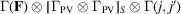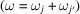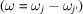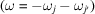International
Tables for
Crystallography
Volume D
Physical properties of crystals
Edited by A. Authier

International Tables for Crystallography (2013). Vol. D, ch. 2.3, pp. 346-347

Section 2.3.6. Higher-order scattering

I. Gregoraa*

aInstitute of Physics, Academy of Sciences of the Czech Republic, Na Slovance 2, CZ-18221 Prague 8, Czech Republic
Correspondence e-mail: gregora@fzu.cz

2.3.6. Higher-order scattering

| top | pdf |

In higher-order processes, the scattering involves participation of two or more quanta (j and) of the elementary excitations. Let us discuss briefly the second-order scattering by phonons, where the energy and wavevector conservation conditions readThe combinations of signs in these equations correspond to four possibilities, in which either both phonons, j and, are created (Stokes process:), both annihilated (anti-Stokes process: −−), or one is created and the other annihilated (difference process:,). If in the Stokes or anti-Stokes case both excitations are of the same type,, one speaks of overtones. The corresponding terms in the transition susceptibility are the coefficients of a bilinear combination of normal coordinates in the expansion of.

In the quasi-static limit, the transition susceptibilities for the second-order scattering correspond, again, to the susceptibility derivatives. Thus, the spectral differential cross section for the second-order scattering (Stokes component) can be formally written aswith. In this formula, we have suppressed the universal factors [see (2.3.3.5)] and the explicit expression for the response function (thermal factors). Instead, the delta function (response function in the limit of zero damping) expresses the energy-conservation condition.

The wavevector selection rules in the long-wavelength limit, with, imply that(the same holds for anti-Stokes components, whilefor difference scattering), so the wavevectors themselves need not be small and, in principle, scattering by phonons with all wavevectors from the Brillouin zone can be observed.

Without invoking any symmetry arguments for the Raman activity, such as the restrictions imposed by crystal symmetry on the susceptibility derivatives, it is clear that the intensity of second-order scattering at a frequencyis controlled by the number of those combinations of phonons whose frequencies obey. The quantity determining this number is the combined density of states of phonon pairs, i.e.This function can be calculated provided the dispersion curvesof the excitations are known. The density of states is a continuous function and shows features known as the van Hove singularities corresponding to the critical points, where one or more components of the gradientvanish. Most of the critical points occur for wavevectors on the boundary, where the vanishing gradients of the individual dispersion curves are often dictated by the crystal symmetry, but they also occur in those regions of the reciprocal space where both dispersion curves have opposite or equal slopes at the same wavevector q. To a first approximation, the second-order spectrum is thus essentially continuous, reflecting the two-phonon density of states, with peaks and sharp features at frequencies close to the positions of the van Hove singularities. This is to be contrasted with the first-order scattering, where (in perfect crystals) only single peaks corresponding to long-wavelength () phonons occur.

Group-theoretical arguments may again be invoked in deriving the selection rules that determine the Raman activity of a particular combination of excitations (Birman, 1974). The susceptibility derivative again transforms as a tensor. For a given pair of excitations () and () responsible for the modulation, the combined excitation symmetry is obtained by taking the direct product of the irreducible representations of the space group corresponding to the participating excitations,The representation, unlike, corresponds to a zero-wavevector representation of the crystal space group and is therefore equivalent to a (reducible) representation of the crystal point group. It can be decomposed into irreducible components. Raman scattering of the pair is allowed if a Raman-activerepresentation is contained in this decomposition ofor, alternatively, if the productcontains the totally symmetric representation.

The selection rules for the second-order scattering are, in general, far less restrictive than in the first-order case. For example, it can be shown that for a general wavevector q in the Brillouin zone there are no selection rules on the participation of phonons in the second-order scattering, since the representationscontain all Raman-active symmetries. In specific crystal structures, however, restrictions occur for the wavevectors corresponding to special symmetry positions (points, lines or planes) in the Brillouin zone. This implies that the selection rules may suppress some of the van Hove singularities in the second-order spectra.

Morphic effects in second-order scattering, due to an applied external force F (see Section 2.3.4.1), may be investigated using the same criteria as in first-order scattering, i.e. decomposing therepresentationand searching for the matrix form of the corresponding second-order Raman tensors.

Generalization to third- and higher-order processes is obvious.

Concluding this section, we note that in a Raman-scattering experiment, higher-order features in the spectra can in principle be distinguished from first-order features by different behaviour of the differential scattering cross section with temperature. For example, the respective thermal factors entering the expression for the second-order scattering cross section are given in Table 2.3.6.1.

 Table 2.3.6.1| top | pdf | Thermal factors for second-order Raman scatteringis given by (2.3.3.7).
FactorProcessRaman shiftStokesDifferenceAnti-StokesReferences

Birman, J. L. (1974). Theory of crystal space groups and infrared and Raman lattice processes of insulating crystals. Handbuch der Physik, Vol. 25/2b. Berlin, Heidelberg, New York: Springer.